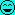# B Calculating pressure with U.S. customary units

#### pvorisek25

Summary
I know that p = F / S. And if I want to calculate pressure in lb/in2 I have to calculate with mass. That means p = (m * g) / S. But that does not work with lb/in2.
(Sorry for my bad English.)

Hello,

I am trying to understand U.S. customary units (don't ask why I am trying to do this) but when I was calculating pressure to lb/in2 I went into problem. I remember that p = F / S but when you have mass you have to multiply mass by gravitational acceleration to get force. So that means p = (m * g) / S. But when I have m = 10 lb; S = 100 in and g = 393 in/s2 and calculate p = (10 * 393) / 100 it just does not work. But when I remove gravitational acceleration it works. But m is mass and we have to multiply it with gravitational acceleration when we want to know force. I searched on Wikipedia and on Wikipedia is that psi isn't pound per square inch but it's pound-force per square inch. But anyway it is still FORCE. Does someone know how it works?

Related Classical Physics News on Phys.org

#### Vanadium 50

Staff Emeritus
Science Advisor
Education Advisor
Pound is a unit for force.

#### Mister T

Science Advisor
Gold Member
In other words, $mg$ equals 10 lbf.

Unfortunately yet another shortcoming of the system is that the pound is officially sanctioned as a unit of mass but is commonly used as a unit of force.

#### pvorisek25

In other words, $mg$ equals 10 lbf.

Unfortunately yet another shortcoming of the system is that the pound is officially sanctioned as a unit of mass but is commonly used as a unit of force.
Oh, I see. Thank you.

#### Anand Sivaram

In imperial units both mass and force are conveniently measured by the unit pound (lb)
1 lb of mass = 0.453 kg, whereas 1lb of force (lbf) = 0.453 * 9.8 = 4.448N = 0.453 (kgf) kg force

Using the same unit for both mass and force are sometimes used in SI and derived units also, considering 1kg of force = 9.80665 N

For example
1 atm pressure = 101325 Pa (N/m2) = 10332 kg force/m2
Considering g = 9.80665
101325 / 9.80665 = 10332

In the same manner torque is measured in ft.lb in Imperial and N.m or Kg.m in SI derived units.

#### vanhees71

Science Advisor
Gold Member
ROTFL about the word "conveniently"#### Mister T

Science Advisor
Gold Member
Using the same unit for both mass and force are sometimes used in SI and derived units also, considering 1kg of force = 9.80665 N
The kilogram-force is not part of SI, and is not sanctioned for use with the SI.

The avoirdupois pound is, by definition, exactly 0.453 592 37 kg.

•vanhees71 and Anand Sivaram

#### Anand Sivaram

Correct. kg-f is not an SI unit. Sorry for the confusion.
The kilogram-force is not part of SI, and is not sanctioned for use with the SI.

#### hutchphd

I was taught that the slug was the official US unit of mass (one slug weighs 32 lbs on earth). I always was amused by the unit.....

#### Chestermiller

Mentor
There are two entities that have units of pounds in Imperial: $lb_f$ and $lb_m$. In addition to the $lb_m$ unit of mass, there is another unit of mass called the slug. When using F = ma, if F is the force in $lb_f$, the correct mass unit to use is the slug. So, $$1\ lb_f=1\ \frac{slug\ ft}{s^2}$$ The $lb_m$ is related to the slug by $$1\ lb_m=1\ \frac{slug}{32.2}$$The $lb_f$ is the force required to accelerate 1 $lb_m$ at the acceleration of gravity, 32.2 ft/s^2. So the $lb_f$ is the weight of 1 $lb_m$. If we want to express Newton's 2nd law in terms of $lb_m$ rather than in terms of slugs, we must write: $$F=\frac{ma}{32.2}$$Hope this helps.

#### jbriggs444

Science Advisor
Homework Helper
There are a number of sets of units that feature something called a "pound".

There is the pound-mass, pound-force, foot, second system in which, as @Chestermiller points out, $F=\frac{ma}{32.2}$ for force in pounds force, mass in pounds mass and acceleration in feet/second2.

There is the slug, pound-force, foot, second system in which $F=ma$ for force in pounds force, mass in slugs and acceleration in feet/second2. The slug is 32.2 pounds mass.

There is the pound-mass, poundal, foot, second system in which $F=ma$ for force in poundals, mass in pounds mass and acceleration in feet/second2. The poundal is 1/32.2 pounds force.

### Want to reply to this thread?

"Calculating pressure with U.S. customary units"

### Physics Forums Values

We Value Quality
• Topics based on mainstream science
• Proper English grammar and spelling
We Value Civility
• Positive and compassionate attitudes
• Patience while debating
We Value Productivity
• Disciplined to remain on-topic
• Recognition of own weaknesses
• Solo and co-op problem solving